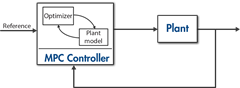# Getting Started with Model Predictive Control Toolbox

Design and simulate model predictive controllers

Model Predictive Control Toolbox™ provides functions, an app, and Simulink® blocks for designing and simulating model predictive controllers (MPCs). The toolbox lets you specify plant and disturbance models, horizons, constraints, and weights. By running closed-loop simulations, you can evaluate controller performance.

You can adjust the behavior of the controller by varying its weights and constraints at run time. To control a nonlinear plant, you can implement adaptive and gain-scheduled MPCs. For applications with fast sample rates, you can generate an explicit model predictive controller from a regular controller or implement an approximate solution.

For rapid prototyping and embedded system implementation, the toolbox supports automatic C-code and IEC 61131-3 Structured Text generation.

## Tutorials

• MPC Modeling

Model predictive controllers use plant, disturbance, and noise models for prediction and state estimation.

• Controller State Estimation

MPC controllers use their current state as the basis for predictions. In general, the controller states are unmeasured and must be estimated.

• Optimization Problem

Model predictive controllers compute optimal manipulated variable control moves by solving a quadratic program at each control interval.

• QP Solver

The model predictive controller QP solver converts an MPC optimization problem to a general form quadratic programming problem.

## VideosWhy Use MPC?
Understand the benefits of using model predictive control.What Is MPC?
Understand the working principles of model predictive control.MPC Design Parameters
Understand common MPC design parameters, such as sample time, horizons, tuning weights, and constraints.